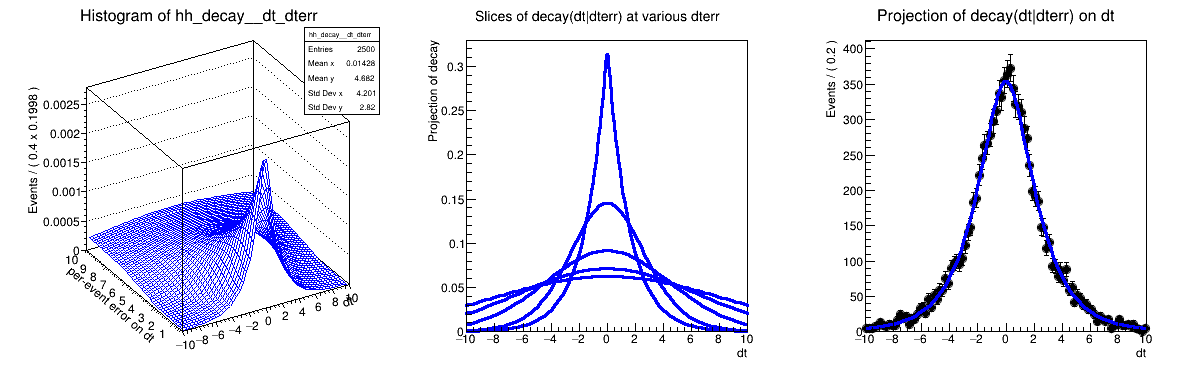# Rf 3 0 6_Condpereventerrors¶

Multidimensional models: complete example with use of conditional pdf with per-event errors

Author: Clemens Lange, Wouter Verkerke (C++ version)
This notebook tutorial was automatically generated with ROOTBOOK-izer from the macro found in the ROOT repository on Saturday, November 28, 2020 at 10:45 AM.

In :
import ROOT

Welcome to JupyROOT 6.23/01


## B-physics pdf with per-event Gaussian resolution¶

Observables

In :
dt = ROOT.RooRealVar("dt", "dt", -10, 10)
dterr = ROOT.RooRealVar("dterr", "per-event error on dt", 0.01, 10)

RooFit v3.60 -- Developed by Wouter Verkerke and David Kirkby
Copyright (C) 2000-2013 NIKHEF, University of California & Stanford University



Build a gaussian resolution model scaled by the per-error = gauss(dt,bias,sigma*dterr)

In :
bias = ROOT.RooRealVar("bias", "bias", 0, -10, 10)
sigma = ROOT.RooRealVar(
"sigma", "per-event error scale factor", 1, 0.1, 10)
gm = ROOT.RooGaussModel(
"gm1", "gauss model scaled bt per-event error", dt, bias, sigma, dterr)


Construct decay(dt) (x) gauss1(dt|dterr)

In :
tau = ROOT.RooRealVar("tau", "tau", 1.548)
decay_gm = ROOT.RooDecay("decay_gm", "decay", dt,
tau, gm, ROOT.RooDecay.DoubleSided)


## Construct fake 'external' data with per-event error¶

Use landau pdf to get somewhat realistic distribution with long tail

In :
pdfDtErr = ROOT.RooLandau("pdfDtErr", "pdfDtErr", dterr, ROOT.RooFit.RooConst(
1), ROOT.RooFit.RooConst(0.25))


## Sample data from conditional decay_gm(dt|dterr)¶

Specify external dataset with dterr values to use decay_dm as conditional pdf

In :
data = decay_gm.generate(ROOT.RooArgSet(


## Fit conditional decay_dm(dt|dterr)¶

Specify dterr as conditional observable

In :
decay_gm.fitTo(data, ROOT.RooFit.ConditionalObservables(
ROOT.RooArgSet(dterr)))

Out:
<cppyy.gbl.RooFitResult object at 0x(nil)>
[#1] INFO:Minization -- RooMinimizer::optimizeConst: activating const optimization
**********
**    1 **SET PRINT           1
**********
**********
**********
PARAMETER DEFINITIONS:
NO.   NAME         VALUE      STEP SIZE      LIMITS
1 bias         0.00000e+00  2.00000e+00   -1.00000e+01  1.00000e+01
2 sigma        1.00000e+00  4.50000e-01    1.00000e-01  1.00000e+01
**********
**    3 **SET ERR         0.5
**********
**********
**    4 **SET PRINT           1
**********
**********
**    5 **SET STR           1
**********
NOW USING STRATEGY  1: TRY TO BALANCE SPEED AGAINST RELIABILITY
**********
**********
FIRST CALL TO USER FUNCTION AT NEW START POINT, WITH IFLAG=4.
START MIGRAD MINIMIZATION.  STRATEGY  1.  CONVERGENCE WHEN EDM .LT. 1.00e-03
FCN=23876.4 FROM MIGRAD    STATUS=INITIATE        8 CALLS           9 TOTAL
EDM= unknown      STRATEGY= 1      NO ERROR MATRIX
EXT PARAMETER               CURRENT GUESS       STEP         FIRST
NO.   NAME      VALUE            ERROR          SIZE      DERIVATIVE
1  bias         0.00000e+00   2.00000e+00   2.01358e-01  -1.70342e+02
2  sigma        1.00000e+00   4.50000e-01   1.63378e-01   8.62474e+01
ERR DEF= 0.5
MIGRAD WILL VERIFY CONVERGENCE AND ERROR MATRIX.
COVARIANCE MATRIX CALCULATED SUCCESSFULLY
FCN=23876.2 FROM MIGRAD    STATUS=CONVERGED      30 CALLS          31 TOTAL
EDM=3.03467e-08    STRATEGY= 1      ERROR MATRIX ACCURATE
EXT PARAMETER                                   STEP         FIRST
NO.   NAME      VALUE            ERROR          SIZE      DERIVATIVE
1  bias         5.26463e-03   1.72103e-02   1.83632e-04   1.00797e-01
2  sigma        9.87130e-01   2.04183e-02   7.70435e-04   2.52620e-03
ERR DEF= 0.5
EXTERNAL ERROR MATRIX.    NDIM=  25    NPAR=  2    ERR DEF=0.5
2.962e-04 -4.402e-06
-4.402e-06  4.169e-04
PARAMETER  CORRELATION COEFFICIENTS
NO.  GLOBAL      1      2
1  0.01253   1.000 -0.013
2  0.01253  -0.013  1.000
**********
**    7 **SET ERR         0.5
**********
**********
**    8 **SET PRINT           1
**********
**********
**    9 **HESSE        1000
**********
COVARIANCE MATRIX CALCULATED SUCCESSFULLY
FCN=23876.2 FROM HESSE     STATUS=OK             12 CALLS          43 TOTAL
EDM=3.12688e-08    STRATEGY= 1      ERROR MATRIX ACCURATE
EXT PARAMETER                                INTERNAL      INTERNAL
NO.   NAME      VALUE            ERROR       STEP SIZE       VALUE
1  bias         5.26463e-03   1.72105e-02   3.67263e-05   5.26463e-04
2  sigma        9.87130e-01   2.04260e-02   3.08174e-05  -9.62778e-01
ERR DEF= 0.5
EXTERNAL ERROR MATRIX.    NDIM=  25    NPAR=  2    ERR DEF=0.5
2.962e-04 -4.786e-06
-4.786e-06  4.172e-04
PARAMETER  CORRELATION COEFFICIENTS
NO.  GLOBAL      1      2
1  0.01361   1.000 -0.014
2  0.01361  -0.014  1.000
[#1] INFO:Minization -- RooMinimizer::optimizeConst: deactivating const optimization


## Plot conditional decay_dm(dt|dterr)¶

Make two-dimensional plot of conditional pdf in (dt,dterr)

In :
hh_decay = decay_gm.createHistogram("hh_decay", dt, ROOT.RooFit.Binning(
50), ROOT.RooFit.YVar(dterr, ROOT.RooFit.Binning(50)))
hh_decay.SetLineColor(ROOT.kBlue)

[#1] INFO:NumericIntegration -- RooRealIntegral::init(gm1_conv_exp(-abs(@0)/@1)_dt_tau_[decay_gm]_Int[dt,dterr]) using numeric integrator RooIntegrator1D to calculate Int(dterr)


Plot decay_gm(dt|dterr) at various values of dterr

In :
frame = dt.frame(ROOT.RooFit.Title(
"Slices of decay(dt|dterr) at various dterr"))
for ibin in range(0, 100, 20):
dterr.setBin(ibin)
decay_gm.plotOn(frame, ROOT.RooFit.Normalization(5.))


Make projection of data an dt

In :
frame2 = dt.frame(ROOT.RooFit.Title("Projection of decay(dt|dterr) on dt"))
data.plotOn(frame2)

Out:
<cppyy.gbl.RooPlot object at 0x7c5b250>

Make projection of decay(dt|dterr) on dt.

Instead of integrating out dterr, a weighted average of curves at values dterr_i as given in the external dataset. (The kTRUE argument bins the data before projection to speed up the process)

In :
decay_gm.plotOn(frame2, ROOT.RooFit.ProjWData(expDataDterr, ROOT.kTRUE))

Out:
<cppyy.gbl.RooPlot object at 0x7c5b250>
[#1] INFO:Plotting -- RooAbsReal::plotOn(decay_gm) plot on dt averages using data variables (dterr)
[#1] INFO:Plotting -- RooDataWeightedAverage::ctor(decay_gmDataWgtAvg) constructing data weighted average of function decay_gm_Norm[dt] over 100 data points of (dterr) with a total weight of 10000
.........................................................................................................................................................................................................................

Draw all frames on canvas

In :
c = ROOT.TCanvas("rf306_condpereventerrors",
"rf306_condperventerrors", 1200, 400)
c.Divide(3)
c.cd(1)
hh_decay.GetZaxis().SetTitleOffset(2.5)
hh_decay.Draw("surf")
c.cd(2)
frame.GetYaxis().SetTitleOffset(1.6)
frame.Draw()
c.cd(3)
frame2.GetYaxis().SetTitleOffset(1.6)
frame2.Draw()

c.SaveAs("rf306_condpereventerrors.png")

Info in <TCanvas::Print>: png file rf306_condpereventerrors.png has been created


Draw all canvases

In :
from ROOT import gROOT
gROOT.GetListOfCanvases().Draw()